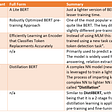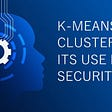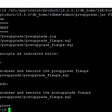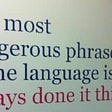# Introduction

In natural language processing, word embedding is used for the representation of words for Text Analysis, in the form of a vector that performs the encoding of the meaning of the word such that the words which are closer in that vector space are expected to have similar in mean.

Consider, boy-men vs boy-apple. Can you tell which of the pairs has more similar words with each other???

For us, it’s obviously easy to understand the associations between words in a language. We know that boy and men have more similar meanings than boy and apple but what if we want machines to understand this kind of association automatically into our languages as well? Then what? Yeah!! That is what word embeddings come into play…

We are going to see the following terms:-

1. Word Embedding Algorithms

2. Library: Gensim

3. Features of Genism Library

4. Word2Vec

5. Implementation

# Word Embedding Algorithms

It is A modern approach to Natural Language Processing.

– Algorithms as word2vec and GloVe have been developed using neural network algorithms.

– Word embedding algorithms provide a Dense Vector Representation of Words that apprehend something about their meaning.

– These algorithms learn about the word by the association the word that is used for.

– A vector space representation of every word, which given by the embedding algorithms provide a projection where words with
similar meanings are locally clustered within the space.

# Library: Gensim

> Gensim is a free Python library designed to automatically extract semantic topics from documents, as efficiently (computer-wise) and painlessly (human-wise) possible.

> Gensim library was developed and is maintained by the Czech digital NLP (natural language processing) scientist Radim Řehůřek and his company named RaRe Technologies.

> Gensim is designed to process raw, unstructured digital texts (“plain text”).

# Features of Gensim Library

Gensim library includes streamed parallelized implementations of the
following:

fastText: This feature uses a neural network for word embedding purposes, which is a library for learning word embedding and text classification as well. The library has developed by the Lab of Facebook AI Research known as FAIR. Basically, this model allows us to create or develop a supervised or unsupervised algorithm to obtain vector representations of words.

word2vec: Word2vec is used to create word embedding which is a also group of shallow and two-layer neural network models. The models are generally trained to reconstruct semantic contexts of words.

doc2vec algorithms: Doc2Vec model is just opposite to the Word2Vec model that is used to develop a vectorized representation of a group of words taken collectively as a single unit. It does not give a simple average of the words in the sentence.

Latent semantic analysis (LSA, LSI, SVD): It is also a technique in NLP (Natural Language Processing) that allows us to analyze relationships between a set of documents and their terms. It is done by constructing a set of concepts related to the documents and terms.

Latent Dirichlet Allocation (LDA): It’s a technique in NLP(Natural Language Processing) that allows sets of observations to be explained by unobserved “groups”. These unobserved groups explain to us why some part is similar of the data. So it is a generative statistical model.

TF-IDF: Term frequency-inverse document frequency is a numeric statistic in information renewal, throwback how important a word is to a document in a corpus. It is frequently used by search engines to score and rank a document’s relevance as per given a user query. It also used for stop-word refining in text summarization and classification.

Formula to calculate tf-idf:-

TF= no of times word occurrences in document/total no of words in the document

IDF= log with base e(total no of documents/no of documents which are having word)

Therefore, tf-idf= TF * IDF

Random projections.

# Word2Vec

Word2vec is a group of models that are used to develop word embeddings.

• Word2vec models are generally shallow, two-layer neural networks that are trained to reconstruct semantic contexts of words.

• Word2vec was created by a team of researchers led by Tomas Mikolov at Google and patented.

• There are two main algorithms on which we can train with Word2Vec namely, CBOW (Continuous Bag of Words) and Skip-Grams.

• We will be using pre-trained algorithms

• Gensim provides the Word2Vec class for working with a Word2Vec model.

# GloVe

GloVe(Global Vectors for Word Representation) is an alternative method to develop word embeddings. It is purely based on matrix factorization techniques on the “word-context matrix”. Normally, we can scan our corpus in the following manner: for every term, we look for context terms within the area defined by a window size before the term and a window size after the term. And hence, we give less amount of weight to more distant words.

Take a look at practical. We will implement a word embedding algorithm here.

# Implementation

Now, we will import the word2Vec library which is an algorithm to perform to learn word associations from given text. Here We have defined data.

`from gensim.models import Word2Vecimport numpy as npsentences = [['drink','not','good'],			['felt','superb'],			['just','good','ambience'],			['bad','taste'],			['parking','problem'],            ['fantastic','food']]y = np.array([0,1,1,0,0,1])`

Let’s train our model with the given data. Also, take a look at the summary of the model.

`model = Word2Vec(sentences, min_count=1,size=100)print(model)`

Now we have the vocabulary, so summarize it which is in the form of a list.

`words = list(model.wv.vocab)print(words)`

Let’s access vector for one word from our word2Vec model.

`print(model['drink'])print(model['fantastic'])`

For the first comment, we are going to find out the mean of embeddings. And then for every data, we will find mean

`means_0 = np.mean(model[sentences],axis=0)means = []for i in sentences :    row_means = np.mean(model[i],axis=0)    means.append(row_means)means = np.array(means) X = means`

Let’s import a random forest classifier from the sci-kit learn library and fit the model accordingly.

`from sklearn.ensemble import RandomForestClassifiermodel_rf = RandomForestClassifier(random_state=1211,                                  n_estimators=100,oob_score=True)model_rf.fit( X , y )test_sentences = [['bad','food'],['just','fantastic']]test_means = []for i in test_sentences :    row_means = np.mean(model[i],axis=0)    test_means.append(row_means)num_test_means = np.array(test_means) X_test = num_test_meansy_pred = model_rf.predict(X_test)`

Now save our word2vec model by using the save function.

`model.save('model.bin')`

If we want to see our model, we will load the word2vec model.

`new_model = Word2Vec.load('model.bin')print(new_model)`

We can also perform PCA(principal component analysis) over the model. And also create a scatterplot of the projection to see our model.

`from sklearn.decomposition import PCAfrom matplotlib import pyplotX = model[model.wv.vocab]pca = PCA(n_components=2)result = pca.fit_transform(X)pyplot.scatter(result[:, 0], result[:, 1])vwords = list(model.wv.vocab)for j, word in enumerate(vwords):pyplot.annotate(word, xy=(result[j, 0], result[j, 1]))pyplot.show()`

At end of this article, we came to the conclusion that how the word embedding approach for representing text is and also how it seems different from other methods like feature extraction. Then you can train a new embedding or can use pre-trained embedding for any natural language processing task!

The following image shows us how a word embedding looks like!

--

--

--

Data Science Student

Love podcasts or audiobooks? Learn on the go with our new app.

## NLP Topic Modeling## Exploring BERT variants (Part 1): ALBERT, RoBERTa, ELECTRA## K-Means Clustering and its Real Use Case in the security domain## Waste Management using Neural Networks## Genetic Algorithms & Evolutionary Machine Learning## Data Spectrometry or How to Preprocess your Data## Using Machine Learning to Detect and Predict the likelihood of a Heart AttackData Science Student

## Oracle 12c to 19c Database Upgrade Manual## Sell more by start thinking you have two markets — local and export## Performing Extract Transform Load (ETL) on Shopee with Phyton## Start Here is the flagship online explainer series for Al Jazeera English (AJE), hosted by Sandra…